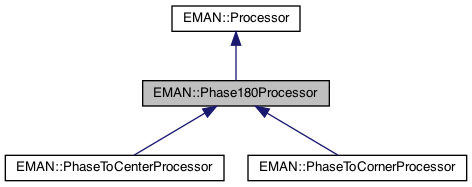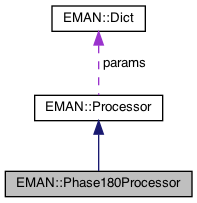EMAN2
EMAN::Phase180Processor Class Reference

This class is abstract. More...

`#include <processor.h>`

Inheritance diagram for EMAN::Phase180Processor:[legend]
Collaboration diagram for EMAN::Phase180Processor:[legend]

## Protected Member Functions

void swap_corners_180 (EMData *image)
swap_corners_180 - works on 2D and 3D images More...

void swap_central_slices_180 (EMData *image)
swap_central_slices_180 - works on 2D and 3D images More...

void fourier_phaseshift180 (EMData *image)
fourier_phaseshift180 - fourier phase shift by 180 this function is called internally if the argument to the process_inplace function is complex. More...Public Types inherited from EMAN::Processor
enum  fourier_filter_types {
TOP_HAT_LOW_PASS , TOP_HAT_HIGH_PASS , TOP_HAT_BAND_PASS , TOP_HOMOMORPHIC ,
GAUSS_LOW_PASS , GAUSS_HIGH_PASS , GAUSS_BAND_PASS , GAUSS_INVERSE ,
GAUSS_HOMOMORPHIC , BUTTERWORTH_LOW_PASS , BUTTERWORTH_HIGH_PASS , BUTTERWORTH_HOMOMORPHIC ,
KAISER_I0 , KAISER_SINH , KAISER_I0_INVERSE , KAISER_SINH_INVERSE ,
SHIFT , TANH_LOW_PASS , TANH_HIGH_PASS , TANH_HOMOMORPHIC ,
}
Fourier filter Processor type enum. More...Public Member Functions inherited from EMAN::Processor
virtual ~Processor ()

virtual void process_inplace (EMData *image)=0
To process an image in-place. More...

virtual EMDataprocess (const EMData *const image)
To proccess an image out-of-place. More...

virtual void process_list_inplace (vector< EMData * > &images)
To process multiple images using the same algorithm. More...

virtual string get_name () const =0
Get the processor's name. More...

virtual Dict get_params () const
Get the processor parameters in a key/value dictionary. More...

virtual void set_params (const Dict &new_params)
Set the processor parameters using a key/value dictionary. More...

virtual TypeDict get_param_types () const
Get processor parameter information in a dictionary. More...

virtual string get_desc () const =0
Get the descrition of this specific processor. More...Static Public Member Functions inherited from EMAN::Processor
static string get_group_desc ()
Get the description of this group of processors. More...

static void EMFourierFilterInPlace (EMData *fimage, Dict params)
Compute a Fourier-filter processed image in place. More...

static EMDataEMFourierFilter (EMData *fimage, Dict params)
Compute a Fourier-processor processed image without altering the original image. More...Protected Attributes inherited from EMAN::Processor
Dict params

## Detailed Description

This class is abstract.

It contains functionality common to the PhaseToCenterProcessor and PhaseToCornerProcessor processors

Date
October 2007

though the testing of this processor is really implicit

Definition at line 6680 of file processor.h.

## ◆ fourier_phaseshift180()

 void Phase180Processor::fourier_phaseshift180 ( EMData * image )
protected

fourier_phaseshift180 - fourier phase shift by 180 this function is called internally if the argument to the process_inplace function is complex.

Parameters
 image the image to be operated upon
Exceptions
 ImageFormatException if the image is not in complex format

Definition at line 6159 of file processor.cpp.

6160{
6161 if ( !image->is_complex() ) throw ImageFormatException("Can not handle images that are not complex in fourier phase shift 180");
6162
6163 // The old code here was seriously broken. It inverted the contrast in some even sized images, and didn't work
6164 // at all for odd images. Ugg. We're calling Pawel's code now. fixed 7/28/18
6165 image->center_origin_fft();
6166
6167// int nx = image->get_xsize();
6168// int ny = image->get_ysize();
6169// int nz = image->get_zsize();
6170
6171// int nxy = nx * ny;
6172
6173
6174// for (int k=-nz/2; k<(nz==1?1:nz/2); k++) {
6175// for (int j=-ny/2; j<ny/2; j++) {
6176// for (int i=(k+j)%2+(j<0?2:0); i<nx/2; i+=2) {
6177// image->set_complex_at(i,j,k,image->get_complex_at(i,j,k)* -1.0f);
6178// }
6179// }
6180// }
6181
6182}
#define ImageFormatException(desc)
Definition: exception.h:147

References ImageFormatException.

## ◆ swap_central_slices_180()

 void Phase180Processor::swap_central_slices_180 ( EMData * image )
protected

swap_central_slices_180 - works on 2D and 3D images

swaps pixels values in central slices, only required if the image has one or more odd dimensions. Should be used striclty in conjunction with swap_central_slices_180 function and never called by anyone except for PhaseToCenterProcessor and PhaseToCornerProcessor classes. Highly specialised function to handle all cases of even and oddness

Parameters
 image the image to be operated upon
Exceptions
 ImageDimensionException if the image is 1D NullPointerException if the image is null

Definition at line 6281 of file processor.cpp.

6282{
6283 int nx = image->get_xsize();
6284 int ny = image->get_ysize();
6285 int nz = image->get_zsize();
6286
6287 int xodd = (nx % 2) == 1;
6288 int yodd = (ny % 2) == 1;
6289 int zodd = (nz % 2) == 1;
6290
6291 int nxy = nx * ny;
6292
6293 float *rdata = image->get_data();
6294
6295 if ( ny == 1 && nz == 1 ){
6296 throw ImageDimensionException("Error, cannot handle 1D images. This function should not have been called");
6297 }
6298 else if ( nz == 1 ) {
6299 float tmp;
6300 if ( yodd ) {
6301 // Iterate along middle row, swapping values where appropriate
6302 int r = ny/2;
6303 for ( int c = 0; c < nx/2; ++c ) {
6304 int idx1 = r*nx + c;
6305 int idx2 = r*nx + c + nx/2+ xodd;
6306 tmp = rdata[idx1];
6307 rdata[idx1] = rdata[idx2];
6308 rdata[idx2] = tmp;
6309 }
6310 }
6311
6312 if ( xodd ) {
6313 // Iterate along the central column, swapping values where appropriate
6314 int c = nx/2;
6315 for ( int r = 0; r < ny/2; ++r ) {
6316 int idx1 = r*nx + c;
6317 int idx2 = (r+ny/2+yodd)*nx + c;
6318 tmp = rdata[idx1];
6319 rdata[idx1] = rdata[idx2];
6320 rdata[idx2] = tmp;
6321 }
6322 }
6323 }
6324 else // nx && ny && nz are greater than 1
6325 {
6326 float tmp;
6327 if ( xodd ) {
6328 // Iterate along the x = nx/2 slice, swapping values where appropriate
6329 int c = nx/2;
6330 size_t idx1, idx2;
6331 for( int s = 0; s < nz/2; ++s ) {
6332 for ( int r = 0; r < ny/2; ++r ) {
6333 idx1 = (size_t)s*nxy+(size_t)r*nx+c;
6334 idx2 = (s+nz/2+zodd)*(size_t)nxy+(r+ny/2+yodd)*(size_t)nx+c;
6335 tmp = rdata[idx1];
6336 rdata[idx1] = rdata[idx2];
6337 rdata[idx2] = tmp;
6338 }
6339 }
6340
6341 for( int s = nz-1; s >= (nz/2+zodd); --s ) {
6342 for ( int r = 0; r < ny/2; ++r ) {
6343 idx1 = (size_t)s*nxy+(size_t)r*nx+c;
6344 idx2 = (s-nz/2-zodd)*(size_t)nxy+(r+ny/2+yodd)*(size_t)nx+c;
6345 tmp = rdata[idx1];
6346 rdata[idx1] = rdata[idx2];
6347 rdata[idx2] = tmp;
6348 }
6349 }
6350 }
6351 if ( yodd ) {
6352 // Iterate along the y = ny/2 slice, swapping values where appropriate
6353 int r = ny/2;
6354 size_t idx1, idx2;
6355 for( int s = 0; s < nz/2; ++s ) {
6356 for ( int c = 0; c < nx/2; ++c ) {
6357 idx1 = (size_t)s*nxy+(size_t)r*nx+c;
6358 idx2 =(s+nz/2+zodd)*(size_t)nxy+(size_t)r*nx+c+nx/2+xodd;
6359 tmp = rdata[idx1];
6360 rdata[idx1] = rdata[idx2];
6361 rdata[idx2] = tmp;
6362 }
6363 }
6364
6365 for( int s = nz-1; s >= (nz/2+zodd); --s ) {
6366 for ( int c = 0; c < nx/2; ++c ) {
6367 idx1 = (size_t)s*nxy+(size_t)r*nx+c;
6368 idx2 = (s-nz/2-zodd)*(size_t)nxy+(size_t)r*nx+c+nx/2+xodd;
6369 tmp = rdata[idx1];
6370 rdata[idx1] = rdata[idx2];
6371 rdata[idx2] = tmp;
6372 }
6373 }
6374 }
6375 if ( zodd ) {
6376 // Iterate along the z = nz/2 slice, swapping values where appropriate
6377 int s = nz/2;
6378 size_t idx1, idx2;
6379 for( int r = 0; r < ny/2; ++r ) {
6380 for ( int c = 0; c < nx/2; ++c ) {
6381 idx1 = (size_t)s*nxy+(size_t)r*nx+c;
6382 idx2 = (size_t)s*nxy+(r+ny/2+yodd)*(size_t)nx+c+nx/2+xodd;
6383 tmp = rdata[idx1];
6384 rdata[idx1] = rdata[idx2];
6385 rdata[idx2] = tmp;
6386 }
6387 }
6388
6389 for( int r = ny-1; r >= (ny/2+yodd); --r ) {
6390 for ( int c = 0; c < nx/2; ++c ) {
6391 idx1 = (size_t)s*nxy+(size_t)r*nx+c;
6392 idx2 = (size_t)s*nxy+(r-ny/2-yodd)*(size_t)nx+c+nx/2+xodd;
6393 tmp = rdata[idx1];
6394 rdata[idx1] = rdata[idx2];
6395 rdata[idx2] = tmp;
6396 }
6397 }
6398 }
6399 }
6400}
#define rdata(i)
Definition: analyzer.cpp:592
#define ImageDimensionException(desc)
Definition: exception.h:166

References ImageDimensionException, and rdata.

## ◆ swap_corners_180()

 void Phase180Processor::swap_corners_180 ( EMData * image )
protected

swap_corners_180 - works on 2D and 3D images

Implements the conventional 180 degree phase shift required to put the center of the image at the bottom left of the image - is used in conjunction with swap_central_slices_180 if any of the image dimensions are odd, but by itself will perform the entire operation on even images. This functions is never called by anyone except for the PhaseToCenterProcessor and PhaseToCornerProcessor classes. Highly specialised function to handle all cases of even and oddness

Parameters
 image the image to be operated upon
Exceptions
 ImageDimensionException if the image is 1D NullPointerException if the image is null

Definition at line 6184 of file processor.cpp.

6185{
6186 int nx = image->get_xsize();
6187 int ny = image->get_ysize();
6188 int nz = image->get_zsize();
6189
6190 int xodd = (nx % 2) == 1;
6191 int yodd = (ny % 2) == 1;
6192 int zodd = (nz % 2) == 1;
6193
6194 int nxy = nx * ny;
6195
6196 float *rdata = image->get_data();
6197
6198 if ( ny == 1 && nz == 1 ){
6199 throw ImageDimensionException("Error, cannot handle 1D images. This function should not have been called");
6200 }
6201 else if ( nz == 1 ) {
6202
6203 // Swap the bottom left and top right corners
6204 for ( int r = 0; r < ny/2; ++r ) {
6205 for ( int c = 0; c < nx/2; ++c) {
6206 int idx1 = r*nx + c;
6207 int idx2 = (r+ny/2+yodd)*nx + c + nx/2+xodd;
6208 float tmp = rdata[idx1];
6209 rdata[idx1] = rdata[idx2];
6210 rdata[idx2] = tmp;
6211 }
6212 }
6213
6214 // Swap the top left and bottom right corners
6215 for ( int r = ny-1; r >= (ny/2+yodd); --r ) {
6216 for ( int c = 0; c < nx/2; ++c) {
6217 int idx1 = r*nx + c;
6218 int idx2 = (r-ny/2-yodd)*nx + c + nx/2+xodd;
6219 float tmp = rdata[idx1];
6220 rdata[idx1] = rdata[idx2];
6221 rdata[idx2] = tmp;
6222 }
6223 }
6224 }
6225 else // nx && ny && nz are greater than 1
6226 {
6227 float tmp;
6228 // Swap the bottom left front and back right top quadrants
6229 size_t idx1, idx2;
6230
6231 for ( int s = 0; s < nz/2; ++s ) {
6232 for ( int r = 0; r < ny/2; ++r ) {
6233 for ( int c = 0; c < nx/2; ++ c) {
6234 idx1 = (size_t)s*nxy+(size_t)r*nx+c;
6235 idx2 = (s+nz/2+zodd)*(size_t)nxy+(r+ny/2+yodd)*(size_t)nx+c+nx/2+xodd;
6236 tmp = rdata[idx1];
6237 rdata[idx1] = rdata[idx2];
6238 rdata[idx2] = tmp;
6239 }
6240 }
6241 }
6242 // Swap the bottom right front and back left top quadrants
6243 for ( int s = 0; s < nz/2; ++s ) {
6244 for ( int r = 0; r < ny/2; ++r ) {
6245 for ( int c = nx-1; c >= (nx/2+xodd); --c) {
6246 idx1 = (size_t)s*nxy+(size_t)r*nx+c;
6247 idx2 = (s+nz/2+zodd)*(size_t)nxy+(r+ny/2+yodd)*(size_t)nx+c-nx/2-xodd;
6248 tmp = rdata[idx1];
6249 rdata[idx1] = rdata[idx2];
6250 rdata[idx2] = tmp;
6251 }
6252 }
6253 }
6254 // Swap the top right front and back left bottom quadrants
6255 for ( int s = 0; s < nz/2; ++s ) {
6256 for ( int r = ny-1; r >= (ny/2+yodd); --r ) {
6257 for ( int c = nx-1; c >= (nx/2+xodd); --c) {
6258 idx1 = (size_t)s*nxy+(size_t)r*nx+c;
6259 idx2 = (s+nz/2+zodd)*(size_t)nxy+(r-ny/2-yodd)*(size_t)nx+c-nx/2-xodd;
6260 tmp = rdata[idx1];
6261 rdata[idx1] = rdata[idx2];
6262 rdata[idx2] = tmp;
6263 }
6264 }
6265 }
6266 // Swap the top left front and back right bottom quadrants
6267 for ( int s = 0; s < nz/2; ++s ) {
6268 for ( int r = ny-1; r >= (ny/2+yodd); --r ) {
6269 for ( int c = 0; c < nx/2; ++c) {
6270 idx1 = (size_t)s*nxy+(size_t)r*nx+c;
6271 idx2 = (s+nz/2+zodd)*(size_t)nxy+(r-ny/2-yodd)*(size_t)nx+c+nx/2+xodd;
6272 tmp = rdata[idx1];
6273 rdata[idx1] = rdata[idx2];
6274 rdata[idx2] = tmp;
6275 }
6276 }
6277 }
6278 }
6279}

References ImageDimensionException, and rdata.

The documentation for this class was generated from the following files: